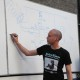## Relatively simple DFT example for dsPIC

I’ve been trying to create a simple DFT example for the dsPIC. I found it more difficult than I expected to come up with something which is both useful and readable. I’m intentionally not doing either of the following:

1. Using the special DSP hardware features of the dsPIC.
2. Using an FFT algorithm.

Obviously, this could run faster if I did either or both of the above items. However, I’m trying to create an example that’s as understandable as possible, rather than one which is as fast as possible.

This example records a 64-sample frame of data from AN0 (analog input channel 0, pin 2) and then calculates the DFT and power spectrum, up to and including the Nyquist frequency. (Since the input data is real, the frequency spectrum values above Nyquist are redundant.) The program then searches through the power spectrum to identify the frequency bin of maximum power. Bin 0 (DC) is exluded, since the input signal will presumably have a large DC component.

```//
// dsPIC DFT Example
// Written by Ted Burke
// Last updated 10-12-2013
//

#include <xc.h>
#include <stdio.h>
#include <libpic30.h>

// Configuration settings
_FOSC(CSW_FSCM_OFF & FRC_PLL16); // Fosc=16x7.5MHz, Fcy=30MHz
_FWDT(WDT_OFF);                  // Watchdog timer off
_FBORPOR(MCLR_DIS);              // Disable reset pin

// Function prototypes

// Window length, N (greater than 4 and a power of 2)
#define N 64

// Twiddle factors (64th roots of unity)
const float W[] = {
1.00000, 0.99518, 0.98079, 0.95694, 0.92388, 0.88192, 0.83147, 0.77301,
0.70711, 0.63439, 0.55557, 0.47139, 0.38268, 0.29028, 0.19509, 0.09801,
-0.00000,-0.09802,-0.19509,-0.29029,-0.38269,-0.47140,-0.55557,-0.63440,
-0.70711,-0.77301,-0.83147,-0.88192,-0.92388,-0.95694,-0.98079,-0.99519,
-1.00000,-0.99518,-0.98078,-0.95694,-0.92388,-0.88192,-0.83146,-0.77300,
-0.70710,-0.63439,-0.55556,-0.47139,-0.38267,-0.29027,-0.19508,-0.09801,
0.00001, 0.09803, 0.19510, 0.29030, 0.38269, 0.47141, 0.55558, 0.63440,
0.70712, 0.77302, 0.83148, 0.88193, 0.92388, 0.95694, 0.98079, 0.99519
};

int main()
{

// Setup UART
U1BRG = 48;            // 38400 baud @ 30 MIPS
U1MODEbits.UARTEN = 1; // Enable UART

// time and frequency domain data arrays
int n, k;                     // time and frequency domain indices
float x[N];                   // discrete-time signal, x
float Xre[N/2+1], Xim[N/2+1]; // DFT of x (real and imaginary parts)
float P[N/2+1];               // power spectrum of x
int a, b;                     // twiddle indices
int to_sin = 3*N/4; // index offset for sin
int k_max;                    // index of max frequency bin
float X_max;                  // power at max frequency bin

while(1)
{
// Record N samples @ 10kHz
for (n=0 ; n<N ; ++n)
{
__delay32(3000); // 100us delay
}

// Calculate DFT and power spectrum up to Nyquist frequency
for (k=0 ; k<=N/2 ; ++k)
{
Xre[k] = 0; Xim[k] = 0;
a = 0; b = to_sin;
for (n=0 ; n<N ; ++n)
{
Xre[k] += x[n] * W[a%N];
Xim[k] -= x[n] * W[b%N];
a += k; b += k;
}
P[k] = Xre[k]*Xre[k] + Xim[k]*Xim[k];
}

// Find and print max amplitude frequency bin
X_max = 0;
for (k=1 ; k<=N/2 ; ++k) if (P[k] > X_max)
{
X_max = P[k];
k_max = k;
}
printf("Max freq bin: %d\n", k_max);
}

return 0;
}

// This function reads a single sample from the specified
// microcontroller is running at 30 MIPS.
// The dsPIC30F4011 has a 10-bit ADC, so the value
// returned is between 0 and 1023 inclusive.
{
ADCHS = channel;          // Select the requested channel
ADCON1bits.SAMP = 1;      // Start sampling
__delay32(30);            // 1us delay @ 30 MIPS
ADCON1bits.SAMP = 0;      // Start Converting
}
```

I saved the program above to a file called “main.c” and compiled it using Microchip’s XC16 C compiler. This is my build script:

```xc16-gcc main.c -mcpu=30F4011 -Wl,--script=p30F4011.gld
if errorlevel 0 xc16-bin2hex a.out
```

By the way, I generated the values for the global array of twiddle factors in the above example using the following short C program (which runs on the PC rather than the dsPIC):

```//
// twiddle.c - Print array of twiddle factors
// Written by Ted Burke
// Last updated 10-12-2013
//
// To compile:
//    gcc twiddle.c -o twiddle.exe
//
// To run:
//    twiddle.exe
//

#include <stdio.h>
#include <math.h>

#define N 64

int main()
{
int n;

for (n=0 ; n<N ; ++n)
{
printf("%8.5lf", cos(n*6.2832/N));
if (n<N-1) printf(",");
if ((n+1)%8==0) printf("\n");
}
return 0;
}
```
This entry was posted in Uncategorized. Bookmark the permalink.

### 5 Responses to Relatively simple DFT example for dsPIC

1.Andres says:

Hello
Do you have any example of a dsPIC FFT implementation using its built-in functions like FFTReal32b or FFTComplex ?

•batchloaf says:

Hi Andres,

I’m afraid I don’t.

Ted

2.Johan Hough says:

Hi Ted, do you have an example of an FFT without the library functions? I am sampling a signal with the adc and need to do an FFT on it and find the peak frequency. I tried using the FFTComplexIP function but to no avail. Any help would be appreciated. I am using a dsPIC33F

•batchloaf says:

Hi Johann,

As it happens, I thought I had an FFT example in C somewhere on this blog, but I guess I don’t because I’ve just searched for it and found nothing. Anyway, the above DFT code calculates precisely the same thing as the FFT, but just not as efficiently. (i.e. the FFT is just a really efficient algorithm for calculating the DFT.) However, if your window length is short there’s not that much difference between the FFT and the plain old brute force method used above.

How many samples is window you want to transform?

Ted

3.Mohamed Saleck Heyin says:

Hello
I want you to help me find the code that can handle the FFT of a signal voltage and electrical current frequency 50Hz I dsPICDEM2 a card on which I want to do this because the application contains dsPIC30F family I dsPIC30F4011 and particularly dspic30f4013